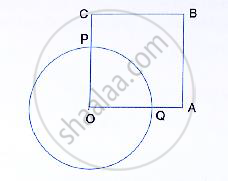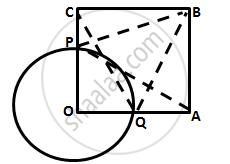Share

In the Following Figure, Oabc is a Square. a Circle is Drawn with O as Centre Which Meets Oc at P and Oa at Q. Prove That: (I) δOpa ≅ δOqc, (Ii) δBpc ≅ δBqa. - ICSE Class 10 - Mathematics

Question

In the following figure, OABC is a square. A circle is drawn with O as centre which meets OC
at P and OA at Q. Prove that:
(i) ΔOPA ≅ ΔOQC, (ii) ΔBPC ≅ ΔBQA.Solution(i)

In ΔOPA and ΔOQC,
OP = OQ          (radii of same circle)
∠AOP = ∠COQ      (both 90°)
OA = OC         (Sides of the square)
By Side – Angle – Side criterion of congruence,
∴ ΔOPA ≅ ΔOQC      (by SAS)

(ii)
Now, OP = OQ (radii)
And OC = OA (sides of the square)
∴ OC – OP = OA – OQ
⇒ CP = AQ          …………… (1)
In ΔBPC and ΔBQA,
BC = BA         (Sides of the square)
∠PCB = ∠QAB       (both 90°)
PC = QA               (by (1))
By Side – Angle – Side criterion of congruence,
∴ ΔBPC ≅ ΔBQA (by SAS)

Is there an error in this question or solution?
Solution In the Following Figure, Oabc is a Square. a Circle is Drawn with O as Centre Which Meets Oc at P and Oa at Q. Prove That: (I) δOpa ≅ δOqc, (Ii) δBpc ≅ δBqa. Concept: Concept of Circles.
S